• CFD, Fluid Flow, FEA, Heat/Mass Transfer

CFD stands for Computational Fluid Dynamics - the branch of mechanical engineering dealing with Fluid Flow and Heat Transfer. Whether you are a fresh graduate with engineering degree or a seasonsed professional with industry and design background, you will find plenty of technical stuffs on CFD analyses and CFD software (both commercial such as FLUENT, CFX and COMSOL as well as open-source Gmsh, OpenFOAM and ParaView. However, one must note that the term CFD is also used in commodity trading where it stands for Contracts for Difference.

How many of these names have you heard of? Click on the names to know more about them!Y-plus: Estimation of first layer height near wall

Estimate height of the 1st layer height of the mesh as per recommended y+ value of the chosen solver, a better guess than choosing an arbitrary value.Here is Excel version: Y-Plus and Boundary Layer Height Estimation using Excel. The default values have been specified to avoid arithmetic errors. Please ensure you specify all the values as per the operating conditions chosen in simulation. The default value of y+ is set = 30, appropriate for most high-Re turbulence models. In case a low-Re turbulence model such as SST is being used, change this value to 1 or maximum 5.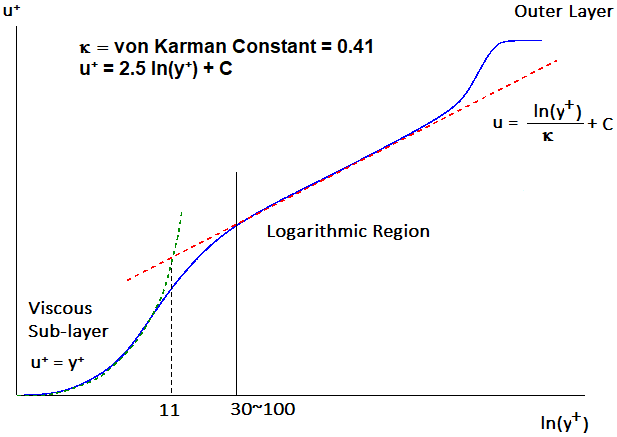Select the type of geometry: - Duct Flow Flat Plate Select the working fluid: [ - ] Air Water Select mode of specifying velocity scale: [ - ] Mean Velocity Volume Flow Rate Mass Flow Rate Specify velocity scale: [m/s] or [m3/s] or [kg/s] Specify cross-section area - ignored if velocity is specified: [m2] Select solver type: 1=Vertex-based (CFX), 2=Cell-centre based (Fluent): [ - ] 1 2 Specify length scale of flow - hyd. dia. (Ducts) or length of the (Flat) plate: [m] Specify working temperature of the fluid: [°C] Specify 'absolute' working pressure (required in case of air only): [bar] Specify desired mean value of y+: [ - ] -

• The calculations are based on empirical correlations and may still require iterations to meet Y+ requirements
• The calculation is based on fully-developed regime. In developing regime, coarse mesh is required and hence the estimated mesh height will hold true for developing region also.
• The calculation is applicable to TURBULENT flows only
• The material properties (viscosity and density) are calculated based on specified fluid (currently available for air and water only) and its temperature.
• The mesh requirements are different for vertex-based formulations (CFX) and cell-centred formulations (Fluent & STAR CCM+)
• For identical mesh, boundary conditions and material properties, CFX will result in Y+ value approx. twice of that in Fluent, if Y+ ≥ 30 everywhere
• If Y+ of flow results in a range 10 ~ 30, the value reported in CFX will not be closer to twice that reported in Fluent. This is because of "scalable" formulation in CFX.
• For 2D geometries, a thickness of 1.0 [m] is assumed to estimate velocity from specified volumetric or mass flow
The steps required to estimate the first layer height is described below:In order to demonstrate the effect of the cell-based and vertex-based scheme, simulations were performed using same mesh, material properties, solver and boundary conditions. The area-averaged value of Y-plus matched the observations described above. The mesh used is shown below.
• The diameter is 100 [mm] and
• the 1st layer height used in CFD simulation is 1.0 [mm]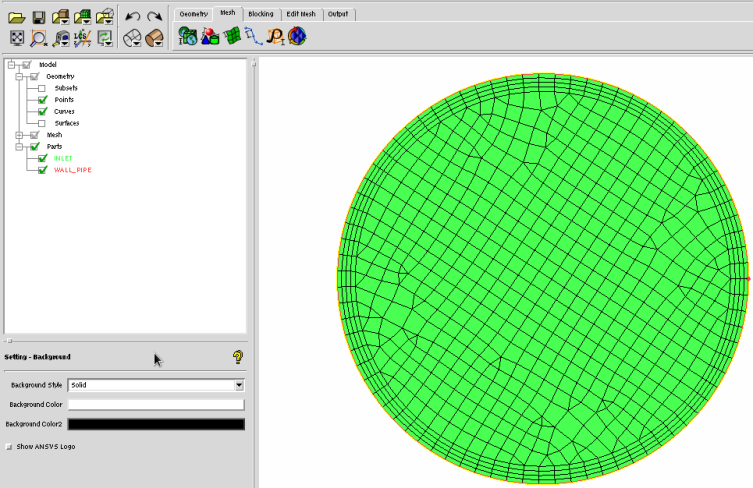The material properties used in CFX and Fluent are described below.The turbulence model used was: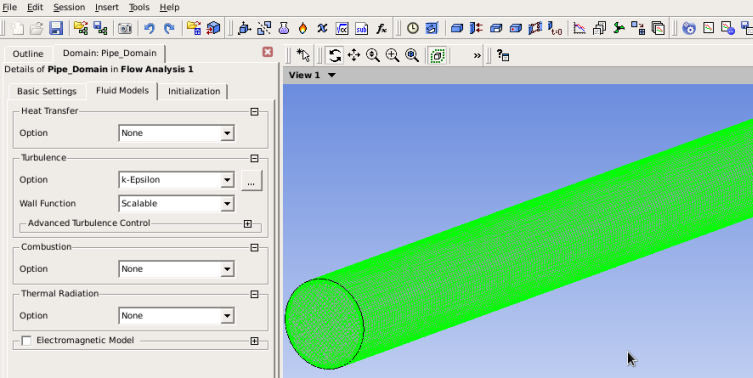The boundary conditions at inlet and outlet are shown below.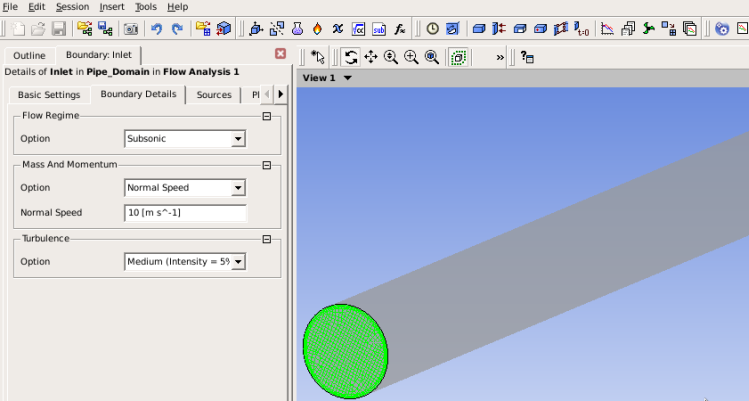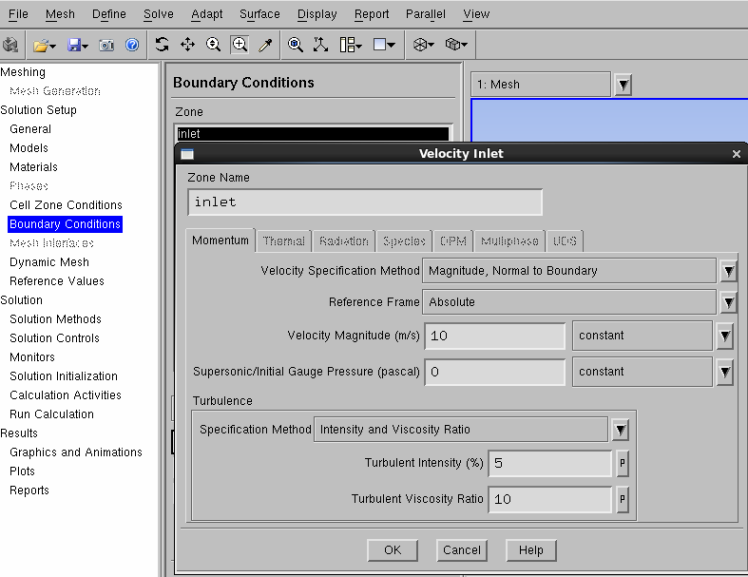A good level of convergence was achieved: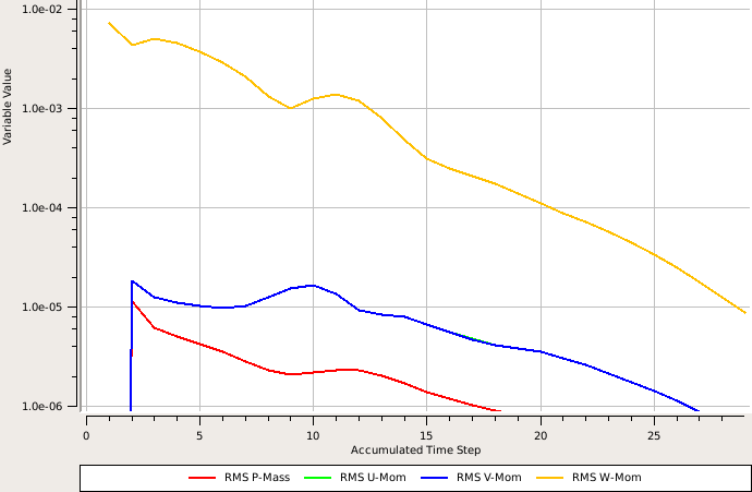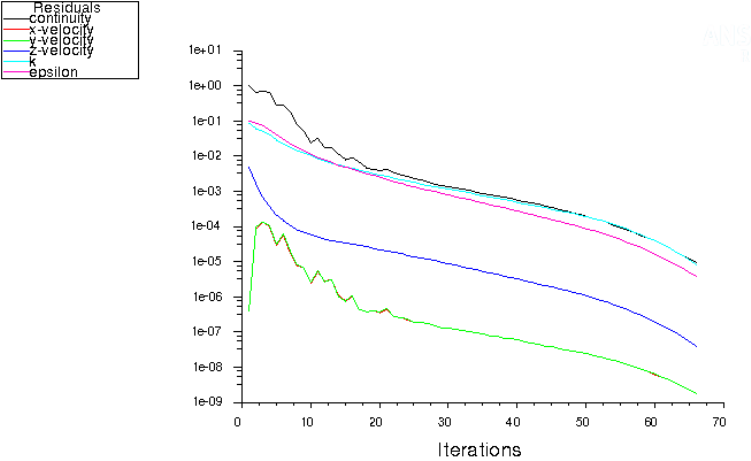## Conclusion:

The contour plots for the Y-plus are shown below. Based on the flow conditions and material properties, following are derived parameters:
• Reynolds number = 65833
• friction factor = 0.0197
• friction velocity = 0.496 [m/s]
• boundary layer height for Y+ = 30 is 0.92 [mm].
• The reported value in CFX with uniform velocity inlet is 33.2.
• The reported value in CFX with uniform velocity inlet is 16.9, which is approximately half of the CFX as expected .
• The reported value in CFX with 7th power velocity profile at inlet is 24.2.
• The Y+ value tend to be higher in the developing region. Hence, Y+ value in flow with uniform velocity inlet is higher than that with velocity profile with power law typically observed in turbulent duct flows.The calculation was repeated in CFX using velocity profile specified by exponential function V0*[1-(r/R)7] keeping all other parameters constant. The reported value of Y-plus has reduced from 33.2 to 24.2.The content on CFDyna.com is being constantly refined and improvised with on-the-job experience, testing, and training. Examples might be simplified to improve insight into the physics and basic understanding. Linked pages, articles, references, and examples are constantly reviewed to reduce errors, but we cannot warrant full correctness of all content.